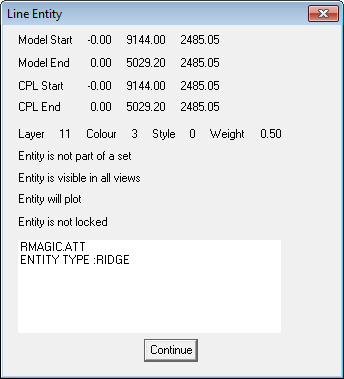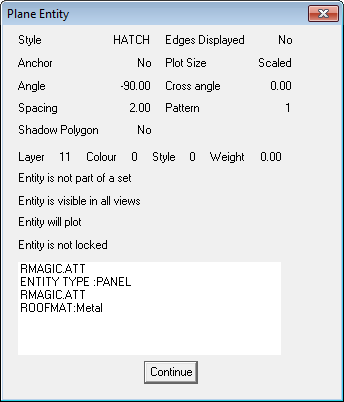# Verify Entity

### Verify Entity

This command reports all the CAD data and information about an entity you select.

The reported information differs depending on the type of entity you have selected.

In the above examples, you will see the tag written on the line making it a RIDGE, and the tag on the plane entity which makes it a PLANE.

Z height of a line.Verify Command 1 – Verifying a line

The model start and model end points are shown for the selected line and the X,Y,Z coordinates for each point is displayed. From this information the Z height of a line, which would be the height of that line above the job datum is displayed. If you were trying to figure the eave height of a roof edge for example, the Z of the start and end points would be the eave height.Verify Command 2 – Verifying a plane

When you select a line the model point coordinates are displayed for the start of the line and the end of the line in the form of X, Y, Z. This is a very useful feature as it will display the actual height of the line relative to the CPL (construction plane) which usually represents the ground level. In effect then, it is giving you the eave height if you select an eave line where the Z height is the eave height.